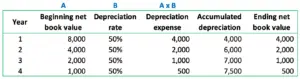# Calculate double declining balance depreciation

## Overview

Double declining balance depreciation is the depreciation method of fixed assets that results in the biggest depreciation expense in the first year and the smallest in the last year of the fixed assets useful life. The company can calculate double declining balance depreciation after determining the estimated useful life of the fixed asset.

The term “double” in the double-declining balance depreciation comes from the determining of deprecation rate to be twice of the straight-line rate. In other words, the depreciation rate in the double-declining balance depreciation method equals the straight-line rate multiplying by two.

Double declining balance depreciation is a type of declining balance depreciation in which the depreciation expense in the early year is bigger than in the later years. In other words, the depreciation expense will reduce from one period to another until the end of the depreciation period where the last depreciation expense is the lowest.

## Double declining balance depreciation

The company can calculate double declining balance depreciation with the formula of the net book value of fixed asset multiplying with the depreciation rate.

Double declining balance depreciation formula:

Double declining balance depreciation = Net book value x Depreciation rate

Net book value can be calculated by using the cost of fixed asst deducting its accumulated depreciation.

Net book value = Cost of fixed asset – Accumulated depreciation.

Depreciation rate in the double-declining balance can be calculated by using the straight-line to multiply with the 2.

Depreciation rate = Straight-line rate x 2

Likewise, the straight-line rate is the rate converted from the useful life of the fixed asset to a percentage figure. For example, if the fixed asset have 4 years of useful life, its straight-line rate can be determined to be 25% per year by using 1 dividing by 4.

## Double declining balance example

For example, the company ABC buys a machine type of fixed asset that costs \$8,000 to use in the business operation. Due to the nature of the machine, the company ABC decides to depreciate it with the double-declining balance depreciation method. The company ABC estimates that the machine has 4 years of useful life with a salvage value of \$500 at the end of its useful life.

Calculate double-declining balance depreciation for the machine above for year 1 to year 4.

Solution:

We have the formula of the double-declining balance depreciation for the fixed assets as below:

Double declining balance depreciation = Net book value x Depreciation rate

Depreciation rate = Straight-line rate x 2

As the machine has 4 years of useful life, the company ABC can determine the straight-line rate to be 25% per year (1 / 4).

In this case, the depreciation rate for the double-declining balance depreciation can be calculated as below:

Depreciation rate = 25% x 2 = 50% per year

Hence, the company ABC can calculate double declining balance depreciation each year for the machine as below:

Year 1:

In the first year, the machine’s net book value at the beginning of the period equals its cost which is \$8,000.

Depreciation expense in year 1 = \$8,000 x 50% = \$4,000

Year 2:

Net book value at the beginning of year 2 = \$8,000 – \$4,000 = \$4,000

Depreciation expense in year 2 = \$4,000 x 50% = \$2,000

Year 3:

Net book value at the beginning of year 3 = \$4,000 – \$2,000 = \$2,000

Depreciation expense in year 3 = \$2,000 x 50% = \$1,000

Year 4:

Net book value at the beginning of year 4 = \$2,000 – \$1,000 = \$1,000

Depreciation expense in year 4 = \$1,000 x 50% = \$500

The four years of depreciation using the double declining balance method can be summarized as in the table below: